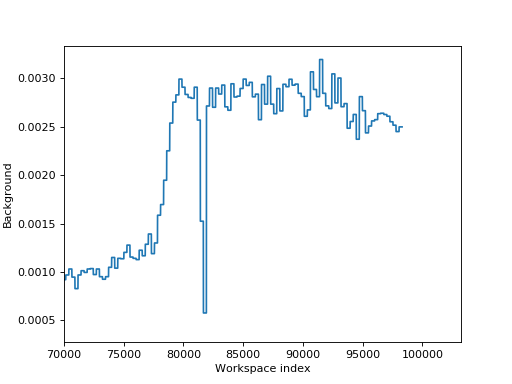$$\renewcommand\AA{\unicode{x212B}}$$

# DirectILLTubeBackground v1¶

## Summary¶

Fits polynomial backgrounds over the pixels of position sensitive tubes.

## Properties¶

Name Direction Type Default Description
InputWorkspace Input MatrixWorkspace Mandatory A workspace to fit the backgrounds to.
OutputWorkspace Output MatrixWorkspace Mandatory The fitted backgrounds.
Components Input str list   A list of component names for which to calculate the backgrounds.
EPPWorkspace Input TableWorkspace Mandatory A table workspace containing results from the FindEPP algorithm.
NonBkgRegionInSigmas Input number 6 Half width of the range excluded from background around the elastic peaks in multiplies of ‘Sigma’ in the EPP table.’.
Degree Input number 0 The degree of the background polynomial.
DiagnosticsWorkspace Input MatrixWorkspace   Detector diagnostics workspace for masking.

## Description¶

This algorithm calculates the time-independent background over instrument components. It is geared towards TOF spectrometers with position sensitive detector tubes such as IN5 or PANTHER at the ILL and can be readily used as a part of ILL’s direct geometry reduction workflow (see here).

The algorithm works as following:

1. Pick a tube from the given list of tube components
2. For each pixel in the tube, calculate the average Y excluding the elastic peak region
3. Fit a polynomial over the averaged Ys of the pixels using CalculatePolynomialBackground
4. Move to the next tube in the list

The OutputWorkspace contains the evaluated background polynomials and can be readily used for background subtraction.

The tube components can be given as a list of component names in Components, or listed as a comma separated string in the instrument parameters file under the name components-for-backgrounds.

The background exclusion range is determined by the EPPWorkspace and NonBkgRegionInSigmas properties. EPPWorkspace is a table workspace containing the centres of the exclusion regions, usually the elastic peaks of a TOF workspace. Such a workspace can be produced for instance by FindEPP or CreateEPP algorithms. The exclusion region is [PeakCentre - NonBkgRegionInSigmas * Sigma, PeakCentre + NonBkgRegionInSigmas * Sigma] where PeakCentre and Sigma are read from EPPWorkspace.

Any pixel for which the FitStatus column in EPPWorkspace is not equal to success is ignored.

A mask workspace produced for example by DirectILLDiagnostics can be given in the DiagnosticsWorkspace property to exclude pixels with known problems. Of course, InputWorkspace can be directly masked, too.

## Usage¶

Note

Example - Usage as part of reduction workflow

from mantid.simpleapi import *
import matplotlib.pyplot as plt
import numpy as np
# Load and preprocess some IN5 data
DirectILLCollectData('ILL/IN5/104007.nxs',
OutputWorkspace='ws', OutputEPPWorkspace='epp')
DirectILLTubeBackground('ws', OutputWorkspace='bkg',
bkg = mtd['bkg']
# Apply the background
ws_bkg_subtracted = Subtract('ws', bkg)
# Plot the background levels of tubes in the forward direction
bkg_y = bkg.extractY()
fig, ax = plt.subplots(subplot_kw={'projection':'mantid'})
xs = np.arange(0, bkg.getNumberHistograms())
ax.plot(xs, bkg_y[:, 0])
ax.set_xlim(xmin=70000)
ax.set_xlabel('Workspace index')
ax.set_ylabel('Background')
# Uncomment the following to show the plot
#fig.show()
# Remove all workspaces
mtd.clear()